# Add Days to Date in MS Excel

If you are an vavid MS Excel user, then you might have come across situations where you need to add the same or different days into the particular date, and if you have done this task manually, then let me add that it’s the most impractical way to do it, especially when MS Excel has the functionality to do it in just a matter of seconds.

So by reading this article carefully, you would get to know different ways to add days into the Date in MS Excel in a few seconds.

So let’s dive into it,

## Ways To Add Days To Date

There are three most excessively used ways to add days to date in MS Excel, stated as follows:

1.    Adding Days to Dates by Value

2.    Adding Days from a specific Cell Location

3.    Adding Days using the DATE() Function

## 1. Adding Days to Dates by Value

Assume that you want to add a predetermined number of days (say, 10) to each date in column A and save the result in column F as shown below: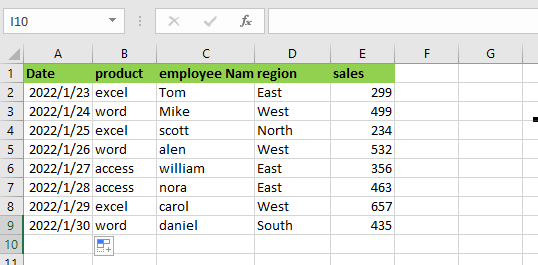So for this, you need to do the steps are given below:

Step1: Please select the first cell of the column where you want to save the result (in our example, cell F2).

Step2: Enter ‘`=`‘ and then choose the first cell in the column holding the dates to which you want to add days (cell A2).

Step3: Then, enter ‘`+`‘ followed by the number of days you wish to add. So, to add 10 days, enter ‘`+10`‘ in the same box. This suggests that the formula for cell F2 should be `=A2+10`.

Step4: On your keyboard, press the Enter In cell F2, you will obtain the result of adding 10 days to the date.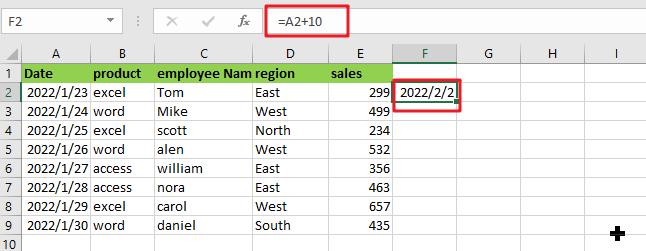Step5: If you want to add the same number of days to all of the dates in Column A, then just pull down the fill handle (at the bottom right of cell F2) to replicate the formula to all of the rows in Column F.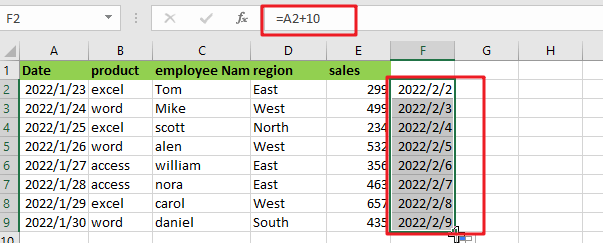## 2.  Adding Days From A Specific Cell Location

If you want to add a different number of days to each date, then put the number of days (which is needed to add) in a separate column for each row (as shown below).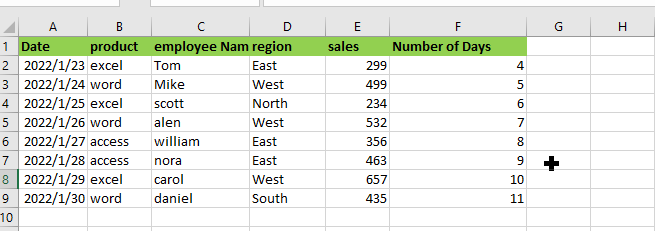Then, follow the instructions outlined below:

Step1: Please select the first cell of the column where you want to save the result (in our example, cell G2).

Step2: Enter `=`‘ and then choose the first cell in the column holding the dates to which you wish to add days (cell A2).

Step3: After that, type `+`‘.

Step4: Then, in our example, choose the first cell in the column showing the number of days to add (cell F2). This suggests that the formula in cell G2 should be `=A2+F2`.

Step5: On your keyboard, press the Enter Add the number of days in cell F2 to the date in cell A2 to get the result.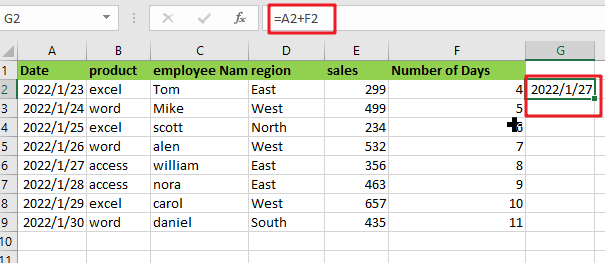Step6: Drag down the fill handle (located at the bottom right of cell G2) to duplicate the formula to all Column G’s rows. This will add the cell in column F to the cell in column A for each row.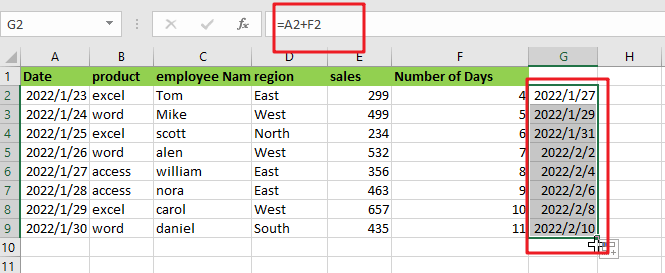Note: Your result may show as a serial number rather than a date in rare situations. This may occur if the format of your result cell is Number or Text. In such circumstances, you may easily convert the serial to a Date format using the following formula:

• Select the cell or the entire column using the right mouse button.
• From the popup menu, choose Format Cells. The Format Cells dialogue box will appear.
• On the Number tab, choose the date from the Category drop-down menu.
• From the Type list on the right side of the dialogue box, choose the format in which you want your date to display.
• Select OK.

This would change the format of all your cells to date.

## 3. Using the DATE() Function to Add Days

There is another method for adding days to a date in Excel. This is accomplished by using the DATE function. Let’s utilize the same dataset as we used before:What you must do is as follows:

Step1: Please select the first cell of the column where you want to save the result (in our example, cell F2).

Step2: Fill up the blanks using the following formula: =DATE(YEAR(A2), MONTH(A2), DAY(A2)+10)

Step3: On your keyboard, press the Enter In cell A2, you will obtain the result of adding 10 days to the date.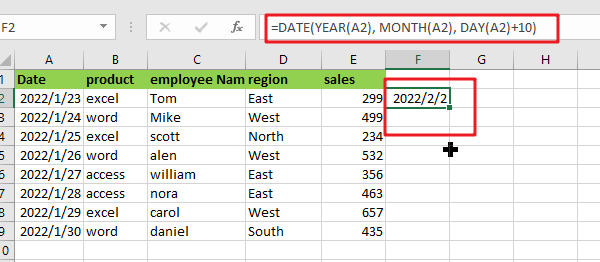Step4: Drag down the fill handle (located at the bottom right of cell F2) to replicate the formula to all of Column F’s rows.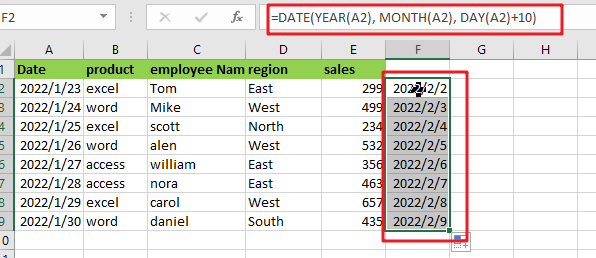You may even add months and years to your date using this approach. Assume you wish to add one year, three months, and four  days to a date. You may simply achieve this using the approach described above.

Simply add 1 to the first parameter, 3 to the second, and 4 to the third, and your formula becomes:

`=DATE(YEAR(A2)+1, MONTH(A2)+3, DAY(A2)+4)`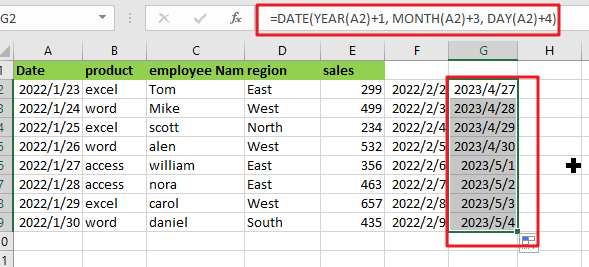### Related Functions

• Excel DAY function
The Excel DAY function returns a day of a date (from 1 to 31).The DAY function is a build-in function in Microsoft Excel and it is categorized as a DATE and TIME Function.The syntax of the DAY function is as below:= DAY (date_value)…
• Excel DATE function
The Excel DATE function returns the serial number for a date.The syntax of the DATE function is as below:= DATE (year, month, day)…
• Excel MONTH Function
The Excel MONTH function returns the month of a date represented by a serial number. And the month is an integer number from 1 to 12.The syntax of the MONTH function is as below:=MONTH (serial_number)…
• Excel YEAR function
The Excel YEAR function returns a four-digit year from a given date value, the year is returned as an integer ranging from 1900 to 9999. The syntax of the YEAR function is as below:=YEAR (serial_number)…